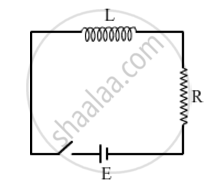# Obtain the Expression for the Magnetic Energy Stored in an Inductor of Self-inductance L to Build up a Current I Through It. - Physics

Obtain the expression for the magnetic energy stored in an inductor of self-inductance L to build up a current I through it.

#### SolutionConsider the circuit shown above consisting of an inductor L and a resistor R, connected to a source of emf E. As the connections are made, the current grows in the circuit and the magnetic field increases in the inductor. Part of the work done by the battery during the process is stored in the inductor as magnetic field energy and the rest appears as thermal energy in the resistor. After sufficient time, the current, and hence the magnetic field, becomes constant and further work done by the battery appears completely as thermal energy. If i be the current in the circuit at time t, we have

E-L(di)/dt=iR

=>Eidt=i^2Rdt+Lidi

=>int_0^tEidt=int_0^ti^2Rdt+int_0^iLidi

=>int_0^t Eidt=int_0^ti^2Rdt+1/2Li^2

Now (idt) is the charge flowing through the circuit during the time t to t+dt. Thus (Eidt) is the work done by the battery in this period. The quantity on the left-hand side of the equation (i) is, therefore, the total work done by the battery in time 0 to t. Similarly, the first term on the right-hand side of equation (i) is the total thermal energy developed in the resistor at time t. Thus

1/2Li^2is the energy stored in the inductor as the current in it increases from 0 to i. As the energy is zero when the current is zero, the energy in an inductor carrying a current i, is U=1/2Li^2

Concept: Solenoid and the Toroid - the Solenoid
Is there an error in this question or solution?